# ML Aggarwal Class 8 Solutions for ICSE Maths Chapter 14 Constructions of Quadrilaterals Ex 14.2

## ML Aggarwal Class 8 Solutions for ICSE Maths Chapter 14 Constructions of Quadrilaterals Ex 14.2

Question 1.
Construct a parallelogram ABCD such that AB = 5 cm, BC = 3·2 cm and ∠B 120°.
Solution: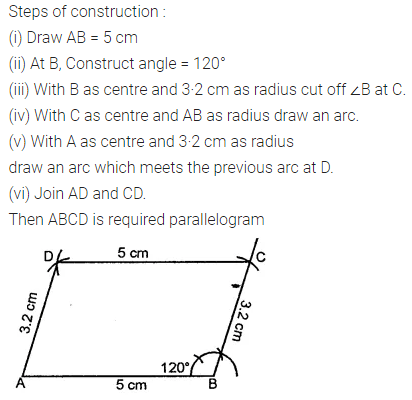Question 2.
Construct a parallelogram ABCD such that AB = 4·8 cm, BC = 4 cm and diagonal BD = 5·4 cm.
Solution: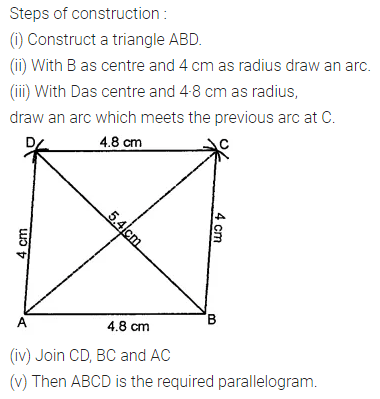Question 3.
Construct a parallelogram ABCD such that BC = 4·5 cm, BD = 4 cm and AC = 5·6 cm.
Solution: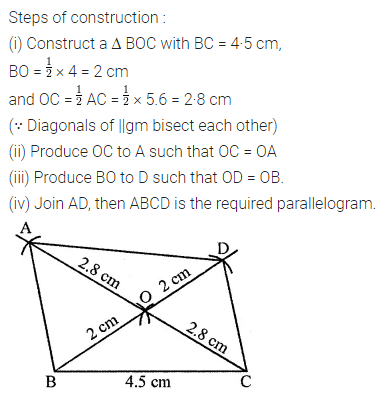Question 4.
Construct a parallelogram ABCD such that AC = 6 cm, BD = 4·6 cm and angle between them is 45°.
Solution:Question 5.
Construct a rectangle whose adjacent sides are 5·6 cm and 4 cm.
Solution:Question 6.
Construct a rectangle such that one side is 5 cm and one diagonal is 6·8 cm.
Solution: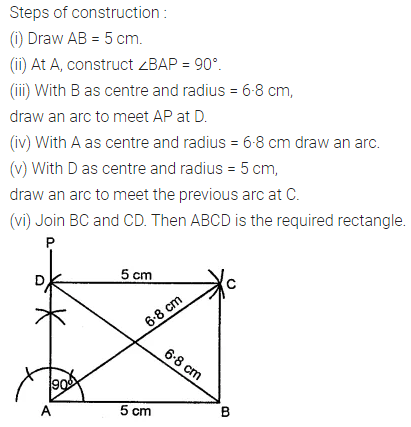Question 7.
Construct a rectangle ABCD such that AB = 4 cm and ∠BAC = 60°.
Solution: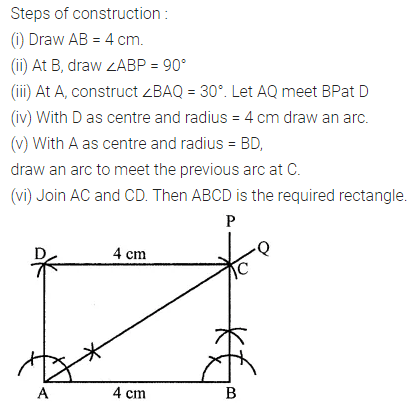Question 8.
Construct a rectangle such that one diagonal is 6-6 cm and the angle between two diagonals is 120°.
Solution:Question 9.
Construct a rhombus whose one side is 5 cm and one angle is 45°
Solution: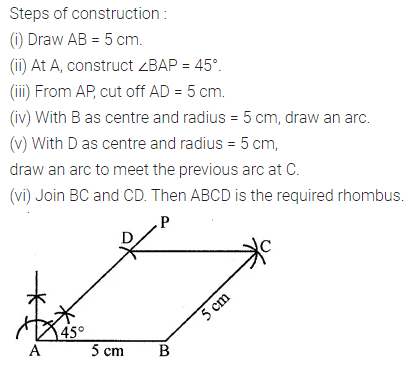Question 10.
Construct a rhombus whose one side is 4·5 cm and one diagonal is 5 cm.
Solution: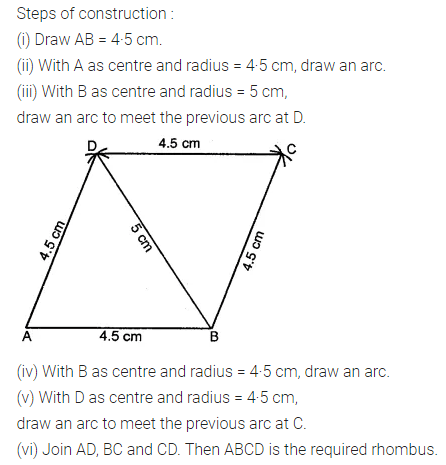Question 11.
Construct a rhombus whose diagonals are 6·8 cm and 5·2 cm.
Solution: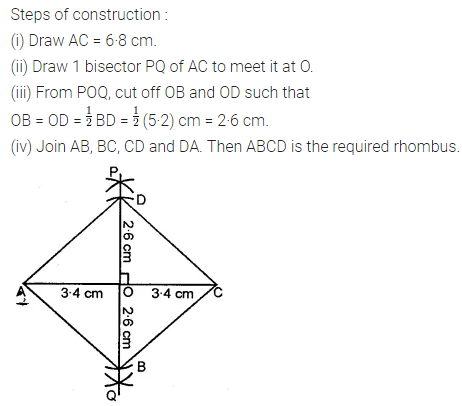Question 12.
Construct a square whose one side is 4·3 cm.
Solution:Question 13.
Construct a square whose one diagonal is 6·2 cm.
Solution: